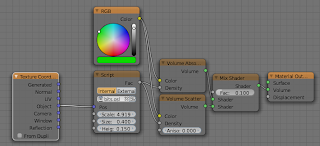### A new tree addon, Part IV: volume rendering experiments with OSL

With the new volume shading options offered by Blenders OSL implementation it is tempting to try this on the trees generated by the space tree addon. Without adding any extra geometry or particles apart form a basic ahape a volume shader might give the impression of a much denser crown as shown in the image below:This effect was achieved by inserting a scaled icosphere that covers about 90% of the crown interior (see below) and adding a volume shader to this icosphere that scatters and absorbs light in a non-uniform manner, i.e. the shader mimics a bunch of small scattered disks, which when seen from the distance add to the illusion of leaves. Note that we cannot do without all the leaves because volume scattering adds no specular reflections as real leaves might do.If you would like to know more about programming OSL you might be interested in my book "Open Shading Language for Blender". More on the availability of this book and a sample can be found on this page.

## Shader code and example node setup

The code for this shader consists of the shader proper, which merely checks which randomly scattered point we are closest to and then calls the `indisk` function with the position of this closest point and a random direction. `indisk` checks whether we are inside a disk with its axis in some direction and returns 1 if this is indeed so. Note that the include at the start of the code refers to a file that is distriubted with Blender and contains a number of useful functions, including a number of Voronoi/Worley related ones.
```#include "node_texture.h"

int indisk(
point p,
point c, float r, float h, vector d
){
vector v = p - c;
float a = dot(v,d);
float lh = abs(length(a * d));
if(lh > h){ return 0;}
float lv = length(v);
float lp = sqrt(lv*lv - lh*lh);
if(lp > r){ return 0;}
return 1;
}

vector randomdirection(point p){
float t = M_2PI*noise("cell",p,1);
float u = 2*noise("cell",p,2)-1;
float s,c,a;
sincos(t,s,c);
a = sqrt(1-u*u);
float x = a*c;
float y = a*s;
float z = u;
return vector(x,y,z);
}

point Pos = P,
float Scale = 1,
float Size = 1,
float Height = 0.05,

output float Fac = 0
){
point p = Pos * Scale;
point centers;
float distances;
voronoi(p, "Distance Squared", 0, distances, centers);
if(indisk(p,centers,Size,Height,randomdirection(p))){
Fac = 1;
}
}```
The node setup to use this shader as seen in the opening image of this article looks like this:## Discussion

Whether using a volume shader this way is really useful remains to be seen: rendering this way is still rather slow. Of course, getting a comparable dense crown with extra particles also slows down rendering: in my tests doubling the number of particles from 1000 to 2000 resulted in a render time that was actually slower than adding the volume shader. In other words, your mileage may vary but it might be worth to experiment.

## References

The space tree addon itself is introduced in a few articles on this blog and is available on GitHub. Relevant links are listed below: